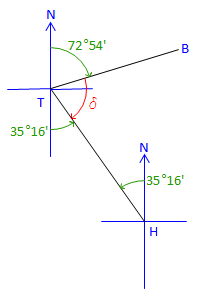## 2. Directions to Angles

Given directions of two adjacent lines, it is a simple matter to determine the angle between the lines.

#### a. Example - Bearings

The bearing of line HT is N 35°16' W , the bearing of line TB is N 72°54' E. What is the angle right at T from B to H?

Sketch:Label the back-direction at T and angle to be computed, δ.Based on the sketch, the desired angle is what’s left over after both bearing angles are subtracted from 180°00'.

δ = 180°00' - (72°54' + 35°16') = 71°50"

#### b. Example - Azimuths

The azimuth of line MY is 106°12', the azimuth of line YF is 234°06'. What is the angle right at Y from F to M?

Sketch:Label the back-azimuth at Y and angle to be computed, ρ.ρ = 286°12' - 234°06' = 52°06'